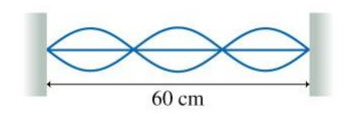# Problem: The figure shows a standing wave oscillating at 100 Hz on a string.What is the wave speed?

###### FREE Expert Solution

Velocity, Wavelength, and frequency are related by

$\overline{){\mathbf{v}}{\mathbf{=}}{\mathbf{f}}{\mathbf{\lambda }}}$

The string has 1.5 wavelengths

85% (198 ratings)###### Problem Details

The figure shows a standing wave oscillating at 100 Hz on a string.What is the wave speed?

Frequently Asked Questions

What scientific concept do you need to know in order to solve this problem?

Our tutors have indicated that to solve this problem you will need to apply the Standing Waves concept. You can view video lessons to learn Standing Waves. Or if you need more Standing Waves practice, you can also practice Standing Waves practice problems.

What professor is this problem relevant for?

Based on our data, we think this problem is relevant for Professor Hayden's class at MSU.